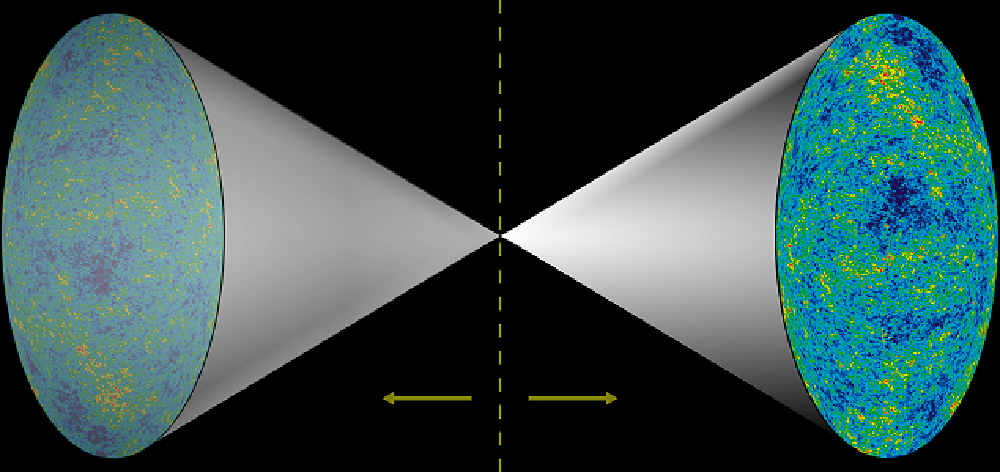# Scenarios (spin 2)

Prime hexagon is a mathematical structure developed by mathematician T. Gallion that is formed when integers are sequentially added to a field of tessellating equilateral triangles, where the path of the integers is changed whenever a prime is encountered.

This is not easy as they are linked to the nature of prime numbers, and nothing is easy about the nature of prime numbers. But I begin with this assumption: if the hexagons participate in the Universe in any way other than haphazardly, they must be demonstrably congruent to something organized (T. Gallion).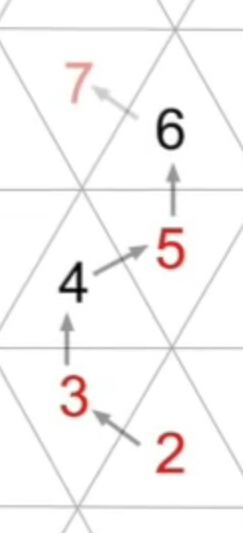This behaviour in a fundamental causal relation to the primes when the products are entered into the partitions system. The subclasses of partitions systemically develops characters similar to the distribution of prime numbers.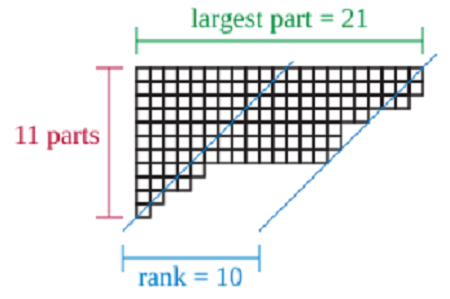Now the following results: Due to the convolution and starting from the desired value of the prime position pairs, the product templates and prime numbers templates of the prime number 7 lie in the numerical Double strand parallel opposite.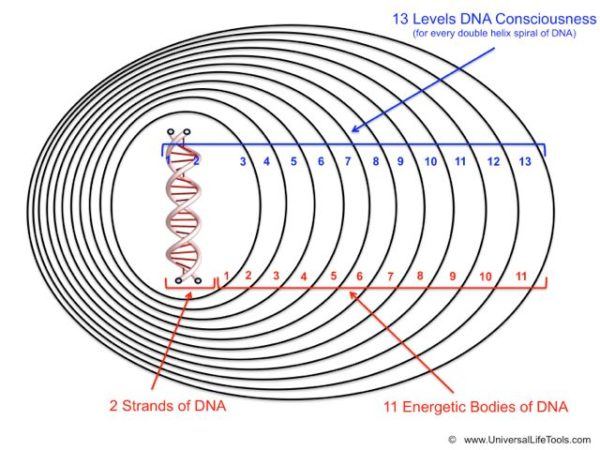The spacetime diagram on the left, the magenta hyperbolae connect events of equal spacelike separation from the origin, while the green hyperbolae connect events of equal timelike separation from the origin.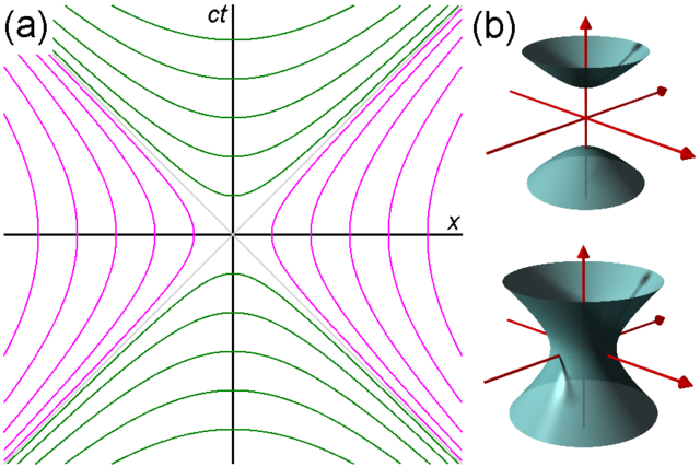This scheme has a configuration of 30 nodes so the recombination is involving 2x30 or 60 nodes out of the 72 nodes of True Prime Pairs will act as the base platform. The rest of 11 which is initially came out from the prime 13 is the irrational.

``````  Tabulate Prime by Power of 10
loop(10) = π(10)-π(1) = 4-0 = 4
loop(100) = π(100)-π(10)-1th = 25-4-2 = 19
loop(1000) = π(1000) - π(100) - 10th = 168-25-29 = 114

-----------------------+----+----+----+----+----+----+----+----+----+-----
True Prime Pairs Δ    |  1 |  2 |  3 |  4 |  5 |  6 |  7 |  8 |  9 | Sum
=======================+====+====+====+====+====+====+====+====+====+=====
19 → π(10)            |  2 |  3 |  5 |  7 |  - |  - |  - |  - |  - | 4th  4 x Root
-----------------------+----+----+----+----+----+----+----+----+----+-----
17 → π(20)            | 11 | 13 | 17 | 19 |  - |  - |  - |  - |  - | 8th  4 x Twin
-----------------------+----+----+----+----+----+----+----+----+----+-----
13 → π(30) → 12 (Δ1)  | 23 | 29 |  - |  - |  - |  - |  - |  - |  - |10th
=======================+====+====+====+====+====+====+====+====+====+===== 1st Twin
11 → π(42)            | 31 | 37 | 41 |  - |  - |  - |  - |  - |  - |13th
-----------------------+----+----+----+----+----+----+----+----+----+----- 2nd Twin
7 → π(60) → 19 (Δ12) | 43 | 47 | 53 | 59 |  - |  - |  - |  - |  - |17th
-----------------------+----+----+----+----+----+----+----+----+----+----- 3rd Twin
5 → π(72) → 18 (Δ13) | 61 | 67 | 71 |  - |  - |  - |  - |  - |  - |20th
=======================+====+====+====+====+====+====+====+====+====+===== 4th Twin
3,2 → 18+13+12 → 43  | 73 | 79 | 83 | 89 | 97 | 101| 103| 107| 109|29th
=======================+====+====+====+====+====+====+====+====+====+=====
Δ                                                            Δ
12+13+(18+18)+13+12   ← 36th-Δ1=151-1=150=100+2x(13+12)   ←   30th = 113 = 114-1

Sequence:
By the next layer the 89² will become 89 and 5 become 5² or 25.
This 89 and 25 are in the same layer with total of 114 or prime 619
So sequence from the first prime is 1,4,7,10,29,68,89,114,139,168,329,618.
``````

When recombination is occur then the prime 13 is forced to → 12 where the impact (Δ1) goes to 18+13+12=43 on the last 7th row. This sequence is simulated by a flowchart having 12 arrows flowing on 10 (ten) shapes of prime 31 up to 71 (40 nodes).

Mapping the quantum way within a huge of primes objects (5 to 19) by lexering (11) the ungrammared feed (7) and parsering (13) across syntax (17) (₠Quantum).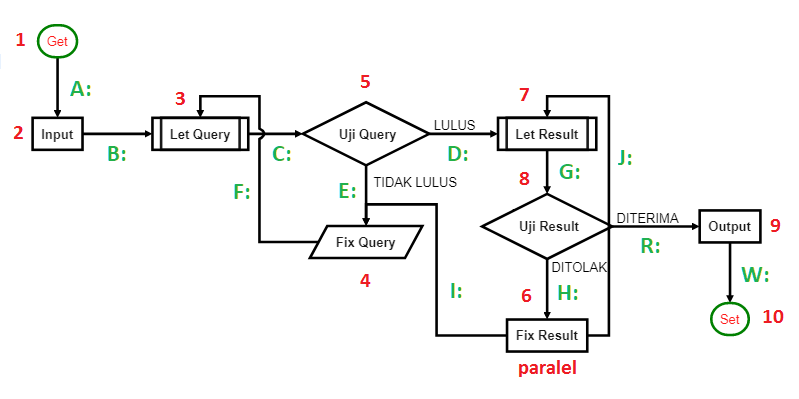This polarity is happened per six (6) cycles by the polar of six (6) to one (1) and six (6) to seven (7) by which we finally found if this behaviour is cascaded bilaterally within the correlation between 61 as the 18th prime and 67 as the 19th prime.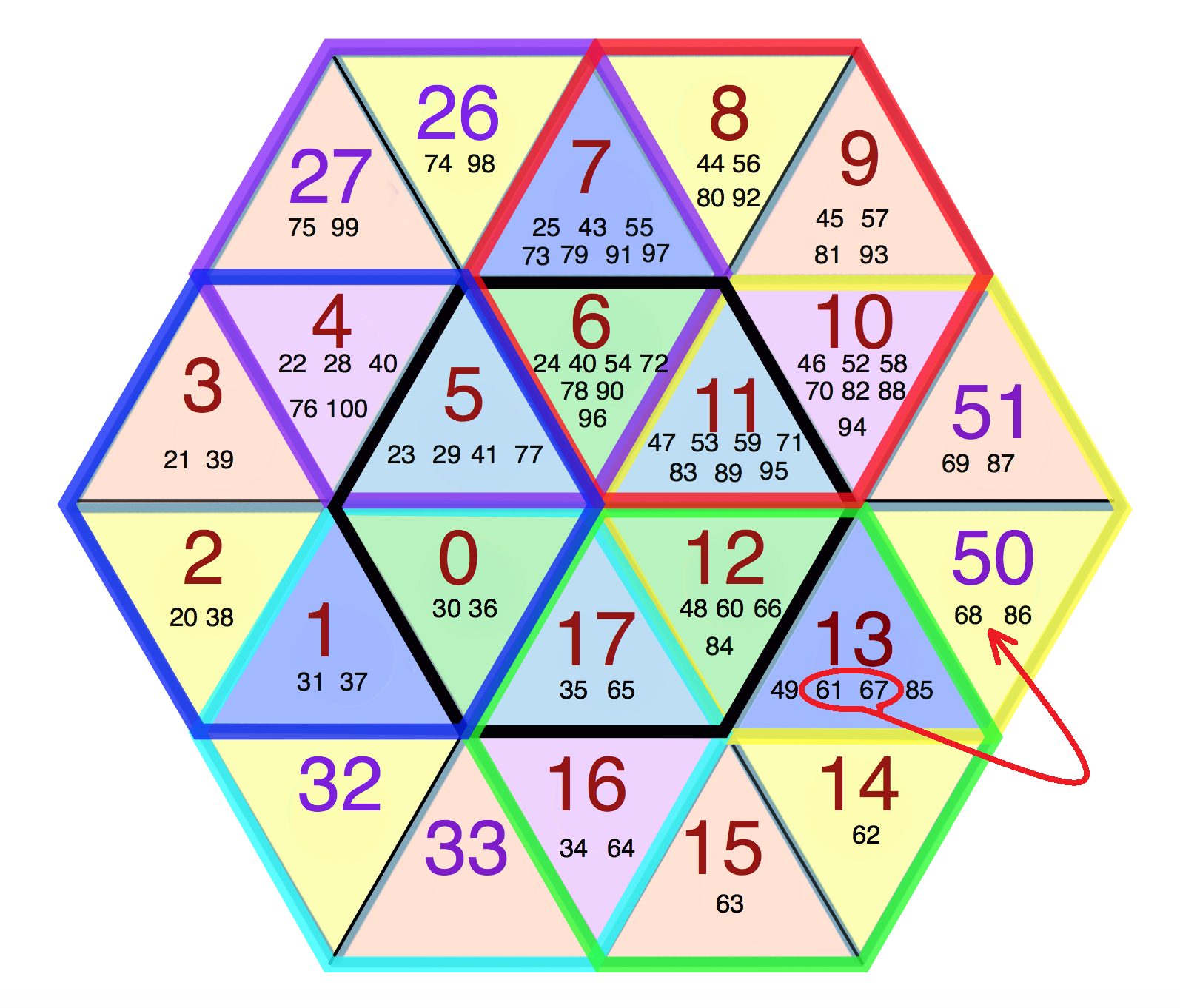Dyson discovered an intriguing connection between quantum physics and Montgomery's pair correlation conjecture about the zeros of the zeta function which dealts with the distribution of primes. This finaly bring us to the equation of Euler's identity.

This scale shows that the Mathematical Elementary Cell 30 (MEC30) standard unites the mathematical and physical results of 1972 by the mathematician Hugh Montgomery and the physicist Freeman Dyson and thus reproduces energy distribution in systems as a path plan more accurately than a measurement (Google Patent DE102011101032A9).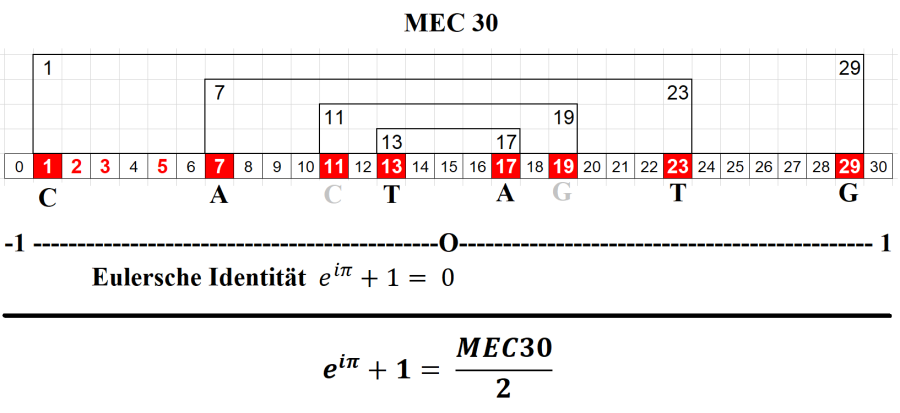The finiteness position of middle zero axis = 15 by the said MEC30 opens up the possibility of accurately representing the self-similarity based on the distribution of True Prime Pairs. So that all number would belongs together with their own identity.